Valence Shell Electron Pair Repulsion Theory

Valence shell electron pair repulsion theory, VSEPR, is a super-simple technique for predicting the shape or geometry of atomic centres in small molecules and molecular ions: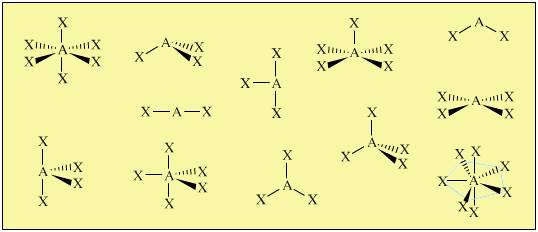Crucially, atomic centres with VSEPR determined geometry can be joined together into molecular entities like cyclohexane and glucose:This molecular building-block logic can be extended, enabling large biomolecular structures like DNA to be modelled and understood:Searches for real chemical entities based on VSEPR geometry can be made using the web based Chemical Thesaurus reaction chemistry database [also by meta-synthesis].

The CoolMolecules Molecular Structure Explorer website classifies molecules by the shape/geometry of the central atom, and all structures are all obtained from experimental data. Searches on the extensive database can be made by atom, shape, experimental method and molecules can be rotated. Highly recommended.

The VSEPR Technique

Six or so steps are required to generate the VSEPR geometry of an atomic centre such as:

• Carbon in methane, CH4
• Nitrogen in ammonia, NH3
• Xenon in xenon tetrafluoride, XeF4
• Iodine in the iodide difluoride ion, [IF2]
 First: Determine the number of electrons in the outer (valence) shell about the central atom (C, N, Xe, I, etc.):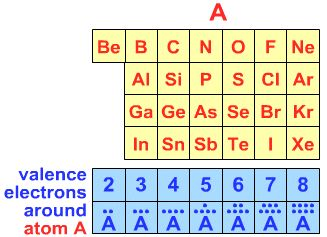Carbon, for example has four valence electrons, nitrogen 5, etc. Second: Find valency and number of electrons associated with the ligand X: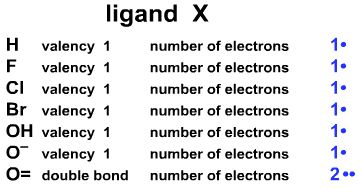Third: Construct a valid Lewis structure of the molecule in question showing all of the bonds and all of the lone pairs (nonbonded pairs) of electrons. If the structure is a molecular ion, add one valence electron for each negative charge and remove one valence electron for each positive charge.Not all Lewis structures have eight electrons about the central atom A (as emphasised by very simple Lewis octet theory). For example, Sulfuric acid, H2SO4, has two monovalent OH functions and two doubly bonded oxygens that behave as single ligands:Phosphorus pentachloride, PCl5, has 10 electrons: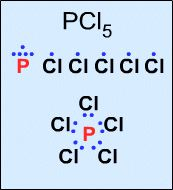Fourth: Determine the "total coordination number" of the central atom, where: total coordination number =                   number of electron pairs =                                     number of electrons in outer shell divided by 2 Methane, CH4, ammonia, NH3, the ammonium ion, [NH4]+ and the nitranion (amide ion), [NH2]–, [above] all have eight electrons in the valence shell of the central atom and all have a total coordination number of 4. Fifth: The overall geometry of the atomic centre is determined by the mutual repulsion between the electron pairs of the total coordination number. The effect can be replicated by holding 2, 3, 4, 5 or 6 balloons together: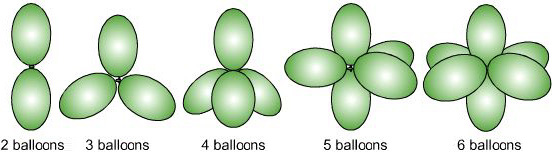2 Balloons give a linear geometry 3 Balloons give a trigonal planar geometry 4 Balloons give a tetrahedral geometry 5 Balloons give a trigonal bipyramidal geometry 6 Balloons give an octahedral geometry Sixth: There two adjustments are required by the VSEPR method to find the geometry of an atomic centre: Lone pairs of electrons (nonbonded pairs) are taken into account in determining the total coordination number and VSEPR geometry, but they are NOT used when defining the geometry of an atomic centre, only the atoms are used: For example, the oxygen of water has two bonded electron pairs (green) and two nonbonded "lone" electron pairs (blue) giving a total VSEPR coordination number of 4. But the geometry is defined by the relationship between the H-O-H atoms and water is said to be "bent" or "angular" shape of 104.5°.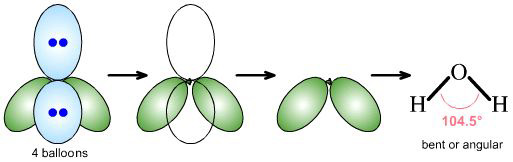Lone-pairs of electrons (blue) behave as if they are slightly bigger than bonded electron pairs (green) and act to distort the geometry about the atomic centre so that bond angles are slightly smaller than expected: Methane, CH4, has a perfect tetrahedral bond angle of 109° 28' (109.47°), while the H-N-H bond angle of ammonia, H3N:, is slightly less at 107°: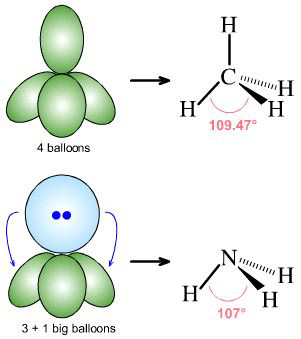By Starjester1 as a Honors Chemistry class project:

The AXE system

American General Chemistry textbooks – but for some reasom not British ones – adopt the excellent AXmEn system, where A is the central atom, m the number of ligands X, and n the number of nonbonded lone-pairs of electrons, E, about the central atom.

In this system:

Methane, CH4, is AX4
Ammonia, H3N:, is AX3E1
water, H2O, is AX2E2

Note that different AXmEn designations can give rise to the same overall geometry or shape:

For example:

AX2E1 and AX2E2 both give rise to bent or angular geometries
AX2 and AX2E3 both give rise to linear geometries

Patterns in AXE Space

The AXE system gives rise to a pattern, from which the various atomic geometric shapes can be determined/assigned: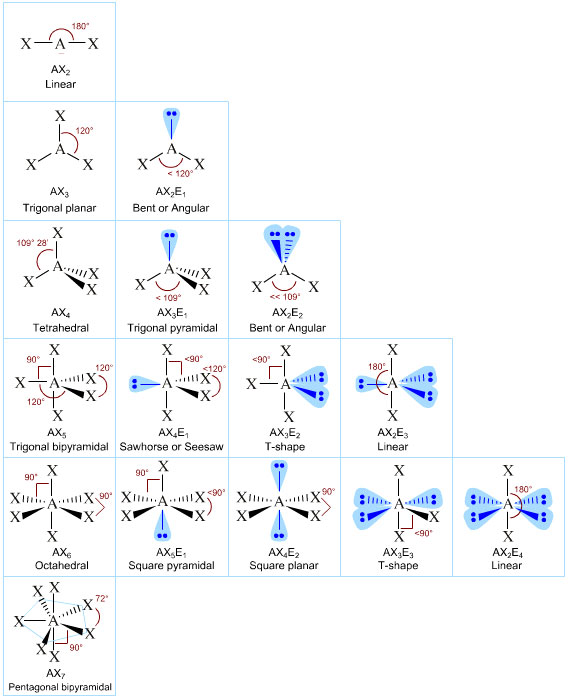A Couple of More Advanced Examples: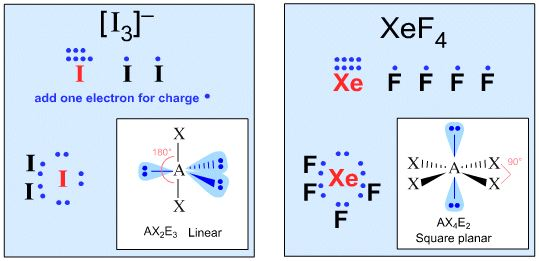Students: What You Need To Know

To be proficient in chemistry at the university entrance level [American AP, British AS/A2 or French Baccalaureate] it is absolutely essential to be able to recognise the VSEPR geometries, know the associated names and work out VSEPR structures from formula of the AEX systems listed below:

AX2
AX3
AX4
AX5
AX6
AX3E1
AX2E2

At university level, all of the atomic centre geometries (plus associated point groups) must be known:

 Linear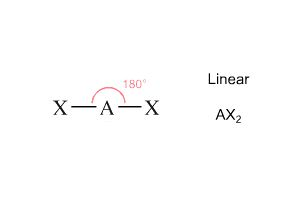Trigonal planar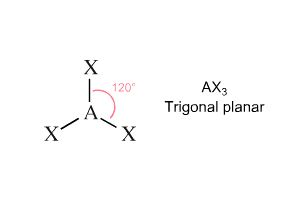Tetrahedral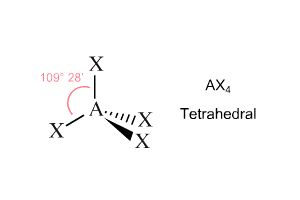Trigonal bipyramidal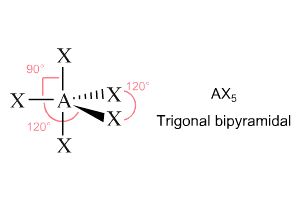OctahedralPentagonal bipyramidal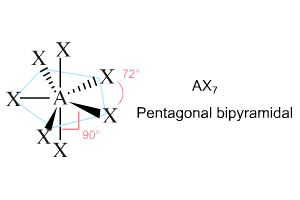Bent or Angular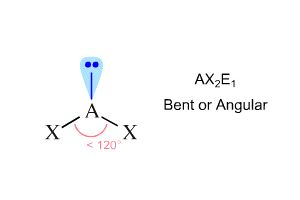Trigonal pyramidal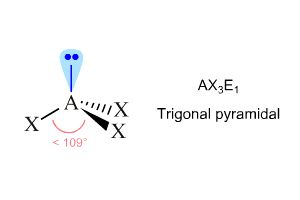Bent or Angular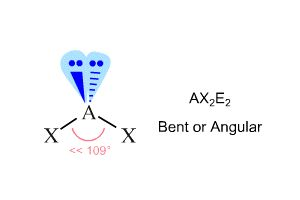Sawhorse or SeesawT-shape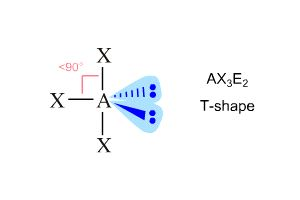Linear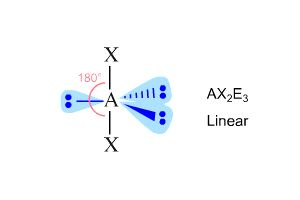Square pyramidal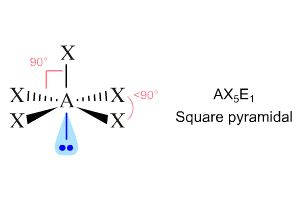Square planar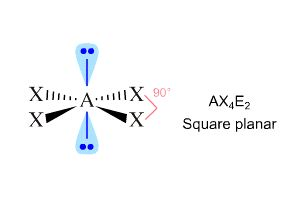T-shape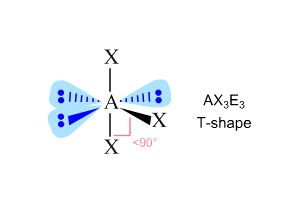Linear© Mark R. Leach 1999 –

If you have any:

Queries